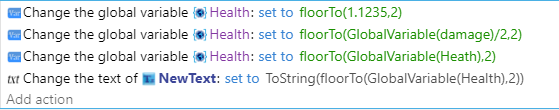# How do you limit a number to two decimal places? Also, what are float and float in range?

To limit a number to two decimal places, do you use FloorTo in the equation? Also, while we’re on the subject, what do float and float in range do?

The wiki “expression reference” is an excellent resource. I keep it linked.

https://wiki.gdevelop.io/gdevelop5/all-features/expressions-reference/

You can use ceilTo(), floorTo() or roundTo()
Round up, down or to nearest nth decimal.

As far as float() and floatInRange(), do you mean randomFloat() and randomFloatInRange() ?

If so, they pick a random floating number either from a default implied 0 to a number or from a number to a number.

2 Likes

Hello Keith. Yes, I use that reference page frequently. I think my question is a bit vague. What I am saying is, if I want to limit my number to only having two decimal places, how do I indicate that in the FloorTo formula? I see that the FloorTo has two numbers. Let’s say I have a GlobalVariable(Health), how do I use that in this formula to limit it to two decimal places? FloorTo(GlobalVariable(Health???

Anywhere you want to reduce it to just a certain number of places you would use one of the expressions with the value or variable and the number of places. I frequently use them when displaying a number in a text object. I’ll keep the variable as it’s full number and then use one of the expressions when setting it to a text object.It behaves like any expression, add the expression anywhere a number is used, put in the value, formula or variable followed by a comma and the number of places.

1 Like

OK perfect. I was suspecting that the # of decimal places was the second number. Thank you.

1 Like

That’s ok. I can see where this can be a bit vague. They are making updates. Hopefully, it will be clearer.